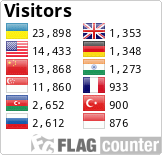DOI: https://doi.org/10.24028/gzh.0203-3100.v41i2.2019.164465

### Mathematical modeling of nonlinear displacement processes in oil LEF-layer by methods of complex analysis and summary representations

#### Abstract

The approach to the modeling of nonlinear displacement processes (one and two-phase filtration) in heterogeneous oil deformable layers is developed, taking into account the inverse effect of the potential of the velocity field and the flow function on the conductivity of the medium. We constructed a method and computational technology for solving the corresponding boundary value problems for nonlinear-layered triple-connected curvilinear domains, bounded by equipotential lines and flow lines, on the basis of the synthesis of numerical methods of quasiconformal mappings and summary representations for differential equations with discontinuous coefficients in combination with domain decomposition by Schwartz method. Quasi-ideal processes in nonlinearly double-layered horizontal LEF-layers, whose geometry of heterogeneity zones is unknown in advance, is described by the corresponding boundary value problems obtained on the basis of the Darcy law and the continuity equation with the coefficient of layer permeability, which is given by a piecewise-constant function with ruptures along the searched equipotentials and lines of flow. The coefficient of conductivity the medium is given as a piecewise-constant function, that is dependent on the searched quasipotential and function of flow, with unknown line dividing layers (lines gap conductance coefficient) that is along the searched equipotential lines and flow lines and that is finding in the process of solving the problem. The proposed algorithms automatically solve the problem of choice of nodes and building a dynamic grid, finding of the unknown dividing lines of areas constancy coefficient of conductivity the medium, the calculation of the velocity field and calculate other characteristic parameters of the process. The decomposition of the domain on the layers of the constancy of permeability coefficient allows us to solve problems in more «comfortable» subdomain than the original problem the whole domain, and allows make in parallel the computational process, since calculations in the subdomain at each iterative step are independent of each other and can be done in parallel with the use of modern computer technology.

#### Keywords

reservoir of oil; doubly-layered medium; quasiconformal mappings; complex quasipotential; summary representations method; domain decomposition; alternating method by Schwarz; LEF-layer

PDF (Українська)

#### References

Bomba, A. Ya., Bulavatskyy, V. M., Skopetskyy, V. V. (2007). Nonlinear mathematical models of processes of heohydrodynamics. Kyiv: Naukova Dumka, 308 p. (in Ukrainian).

Bomba, A. Ya., & Hladka, O. M. (2013). Mathematical modelling of nonlinear filtration processes in shale layers. Fizyko-matematychne modelyuvannya ta informatsiyni tekhnolohiyi, (18), 32—42 (in Ukrainian).

Bomba, A. Ya., Hladka, O. M., & Kuzmenko, A. P. (2016). Computational technologies based on the methods of complex analysis and summary representations. Rivne: Assol, 283 p. (in Ukrainian).

Bomba, A. Ya., Hladka, O. M., & Kuzmenko, A. P. (2014). Methods of complex analysis and summary representations modeling nonlinear processes displacement for the system of two wells in doubly-layered reservoir of oil and gas. Visnyk Ternopilskoho natsionalnoho tekhnichnoho universytetu, (1), 238—251 (in Ukrainian).

Bomba, A. Ya., Kashtan, S. S., Pryhornytskyy, D. O., & Yaroschak, S. V. (2013а). Methods of complex analysis. Rivne: Publ. of the National University of Water Management and Nature Management, 415 p. (in Ukrainian).

Bomba, A. Ya., Kuzmenko, A. P., & Hladka, O. M. (2012). The syntheses of the numeric methods conformal mappings and summary representations in modeling by ideal fields for curvilinear domains. Visnyk Kyyivskoho natsionalnoho universytetu imeni Tarasa Shevchenka. Seriya “Fizyko-matematychni nauky’, (2), 87—94 (in Ukrainian).

Bomba, A. Ya., Yaroshchak, S. V. & Sinchuk, A. M. (2013б). The method of complex analysis of the study of two-phase filtration in horizontal layers, taking into account hydraulic fracturing, Elektronnoye modelirovaniye, (2), 25—33 (in Russian).

Vasilevskiy, Yu. V., & Olshanskiy, M. A. (2007). Short course on multigrid methods and domain decomposition methods. Moscow: Moscow University Press, 105 p. (in Russian).

Hladka, O. M. (2016). Systematic approach to mathematical modeling of filtration processes in multiply-connected curvilinear LEF-layers. Systemni doslidzhennya ta informatsiyni tekhnolohiyi, (2), 58—73. http://dx.doi.org/10.20535/SRIT.2308-8893.2016.2.06 (in Ukrainian).

Lyashko, I. I., & Velikoivanenko, I. M. (1973). Numerical-analytical solution of boundary value problems of filtration theory. Kiev: Naukova Dumka, 264 p. (in Russian)

Sergienko, I. V., Skopetskiy, V. V., & Deyneka, V. S. (1991). Mathematical modeling and research of processes in heterogeneous media. Kiev: Naukova Dumka, 432 p. (in Russian).

Bomba, A. Ya., & Hladka, O. M. (2017). Problems of identification of the parameters of quasiideal filtration processes in nonlinear layered porous media. Journal of Mathematical Sciences, 220(2), 213—225. doi: 10.1007/s10958-016-3178-2.

Bomba, A. Ya., & Hladka, E. N. (2014). Methods of complex analysis of parameters identification of quasiideal processes in nonlinear doubly-layered porous pools. Journal of Automation and Information Sciences, 46(11), 50—62. doi: 10.1615/JAutomatInfScien.v46.i11.60.

Bomba, A. Ya., & Yaroshchak, S. V. (2012). Complex approach to modeling of two-phase filtration processes under control conditions. Journal of Mathematical Sciences, 184(1), 56—68.

Bomba, A. Ya., Yaroshchak, S. V., & Myslyuk, M. A. (2013). Mathematic modelling of thermodynamic effects in a gas formation well bore zone. Journal of Hydrocarbon Power Engineering, (1), 1—4.

Hladka, О., &Bomba, A. (2014). The complex analysis method of numerical identification of parameters of quasiideals processes in doubly-connected nonlinear-layered curvilinear domains. Journal of Mathematics and System Science, 4(7), 514—521. doi: 10.17265/2159-5291/2014.07.009.

Polozhii, G. N. (1965). The method of summary representations for numerical solution of problems of mathematical physics. London: Pergamon Press, 283 p.

#### GOST Style Citations